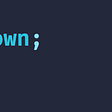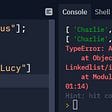# [object Object] Things You Didn’t Know About valueOf

`var obj = {};console.log( 7 + obj ); // “7[object Object]”`
`var obj = {  valueOf: function() {    return 42;  }};console.log( 7 + obj ); // 49`

# Who cares?

`function Int( val ) {  if ( !( this instanceof Int ) ) {    return new Int( val );  }  if ( isNaN( val ) ) {    throw new TypeError(‘Int value must be a number’);  }  if ( val % 1 !== 0 ) {    throw new TypeError(‘Int value must be an integer’);  }  if ( val instanceof Int ) {    return Int( val._value );  }  this._value = Math.floor( val );}Int.prototype.add = function( n ) {  return Int( this._value + Int( n )._value );};Int.prototype.subtract = function( n ) {  return Int( this._value — Int( n )._value );};Int.prototype.multiply = function( n ) {  return Int( this._value * Int( n )._value );};Int.prototype.divide = function( n ) {  try {    return Int( this._value / Int( n )._value );  } catch ( e ) {    throw new Error(‘Division resulted in non-integer quotient’);  }};`
`Int( 6 ).multiply( 2 );        // { _value: 12 }Int( 4 ).divide( 2 ).add( 3 ); // { _value: 5 }`
`var a = Int( 6 );var b = Int( 11 );Math.min( a, b ); // NaN`
`Int.prototype.valueOf = function() { return this._value;};`
`var a = Int( 6 );var b = Int( 11 );Math.min( a, b ); // 6`
`Math.pow( Int( 9 ), 0.5 ); // 3`

--

--

--

## More from Kevin Ennis

Director of Engineer and founding team member @StarryInternet. Formerly @Aereo. @kevincennis on Twitter and Github. http://kevvv.in

Love podcasts or audiobooks? Learn on the go with our new app.## Kevin Ennis

Director of Engineer and founding team member @StarryInternet. Formerly @Aereo. @kevincennis on Twitter and Github. http://kevvv.in

## Let's talk about a couple of Special types## HashMap: Map vs Object in JavaScript## Software engineering principles, Best practices and Introduction to JavaScript## Mutability & Reassignment in JavaScript